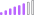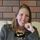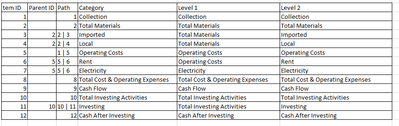cancel
Showing results for
Did you mean:

Fabric is Generally Available. Browse Fabric Presentations. Work towards your Fabric certification with the Cloud Skills Challenge.Post Patron

## One measure formula for a big hierarchy matrix table

Hello!

I'm working on a cash flow report and I made a big hierarchy in order to have a good display on the report. I'm using a matrix table to display the hierarchy with the dates along with the values.

My sample data set is like this:

 Ref Code Code Category Parent Category Identifier for formula Date Amount 32435 GUJS5 Collection Collection Operating1 Jan-20 5763 353523 3RER Collection Collection Operating1 Feb-20 5764 4646 FET4 Imported Total Materials Operating2 Mar-20 5765 3634 VRW3 Imported Total Materials Operating2 Apr-20 5766 3535 FET48 Local Total Materials Operating3 May-20 5767 46456 DW3 Electricity Operating Costs Operating4 Jan-20 5768 2646 D3F6 Rent Operating Costs Operating5 Jan-20 5769 263 D3R Rent Operating Costs Operating5 May-20 5770 264 HUHU56 Rent Operating Costs Operating5 Feb-20 5771 265 JDI3 Local Total Materials Operating3 May-20 5772 266 ECE6 Collection Collection Operating1 Feb-20 5773 267 CFHU12 Imported Total Materials Operating2 May-20 5774 268 GHUIKJSB64 Investing Total Investing Activities Investing1 Jan-20 5775 269 HUH3 Investing Total Investing Activities Investing1 May-20 5776 270 HBP7 Investing Total Investing Activities Investing1 Feb-20 5777 271 BIO5 Investing Total Investing Activities Investing1 May-20 5778

My Hierarchy Data on a different table (DAX table) is similar to this:

 Item ID Parent ID Path Category 1 1 Collection 2 2 Total Materials 3 2 2 | 3 Imported 4 2 2 | 4 Local 5 1 | 5 Operating Costs 6 5 5 | 6 Rent 7 5 5 | 6 Electricity 8 8 Total Cost & Operating Expenses 9 9 Cash Flow 10 10 Total Investing Activities 11 10 10 | 11 Investing 12 12 Cash After Investing

And my hierarchy on a matrix table looks like this:

• Collections
• Total Materials
• Imported
• Local
• Operating Costs
• Rent
• Electricity
• Total Costs & Operating Expenses (Total Materials + Operating Costs)
• Cash Flow (Total Costs & Operating Expenses + Collections)
• Total Investing Activities
• Investing
• Cash After Investing (Cash Flow + Total Investing Activities)

I made the hierarchy that way for easy view on user (since this is the cashflow). In order to have a clean matrix table. I planned to have a single column/measure for the amounts. However, I don't know how to approach for the amounts of the hierarchy columns that has specific formulas.

The following is my plan that I can't seem to execute (a different approach would also be helpful):

Amount (measure)

If parent category is Collections: sum(collections)

If parent category is Total Materials: sum(total materials)

If parent category is Total Costs & Operating Expenses: Total Materials + Operating Costs

If parent category is Cash Flow: Total Costs & Operating Expenses + Collections

If parent category is Cash After Investing: Cash Flow + Total Investing Activities

and so on and so forth.

How should I compute for the Amounts? I'm really stucked at this and any help is appreciated.

Thank you!

16 REPLIES 16Super User

@crln-blue  glad you're making some progress. Keep me updated on how it goes. Share an updated file if you want me to have another look. I might need to take the weekend off though. 😃

Copying DAX from this post? Click here for a hack to quickly replace it with your own table names

Has this post solved your problem? Please Accept as Solution so that others can find it quickly and to let the community know your problem has been solved.

I work as a Microsoft trainer and consultant, specialising in Power BI and Power Query.
www.excelwithallison.comSuper User

@crln-blue Thanks, that file was super helpful. You have done some good troubleshooting already - looks like the ISINSCOPE isn't behaving as we need it to. I believe this is due to the fact that you're trying to do a self join on Table 2. If you look at Page 2 of the attached file (below signature) you will see I have started making separate tables and these behave better with the ISINSCOPE function (I suggest you create these tables in Power Query not DAX, but you have already created many DAX columns, so I just used the DAX table you supplied to save time - recreate path, etc in Power Query).

That will probably solve alot of your problems, but then the other issue is trying to return a value for a category that is not in scope - so you need some ALL and other filter functions. Have a look at how I have started changing your IF-ISINSCOPE measure to see what I mean.

Copying DAX from this post? Click here for a hack to quickly replace it with your own table names

Has this post solved your problem? Please Accept as Solution so that others can find it quickly and to let the community know your problem has been solved.

I work as a Microsoft trainer and consultant, specialising in Power BI and Power Query.
www.excelwithallison.comPost Patron

Hi @AllisonKennedy ! Thank you very much for checking the file. I'm not familiar with ALL and ALLEXCEPT functions (currently learning now) but I noticed something. The subtraction from another category isn't generating the expected result. It seems like it's just taking the sum of the first category and didn't subtract at all (Category Diff). Whereas, for the subtraction in another category (B), it did subtract as expected. I tried changing the formula for the Category Diff similar to category B but I think it gave a blank value since it suddnely disappeared from the matrix. Also, what's the 10 for?

Thanks for all the help!Super User

@crln-blue  You can remove the 10, that was just me testing to see if it would return the value or blank. I was struggling a bit with your Category Diff, think it might have been due to the self join, so are you using the new model with multiple tables or still one table? Also, the tricky part about Category Diff is that you're trying to access values not within that category at all, wheres "b" is looking for 'c' - 'd' which are all in the same Level 1 category (I'm writing this from memory so sorry if I mixed up some names, but hopefully it makes sense).

Copying DAX from this post? Click here for a hack to quickly replace it with your own table names

Has this post solved your problem? Please Accept as Solution so that others can find it quickly and to let the community know your problem has been solved.

I work as a Microsoft trainer and consultant, specialising in Power BI and Power Query.
www.excelwithallison.comPost Patron

Hello @AllisonKennedy ,
I'm trying to adjust my tables into separate tables for items and categories. I managed to get the difference but I can't link back the calculations of the parents' categories to the main hierarchy. I'll also take your advice and try to put the needed child categories for the category differences.Post Patron

Hi @AllisonKennedy , link can now be accessed. For some reason, my replies aren't showing here.

Here's my IFISINSCOPE calculation which shows blank values for addition, subtraction:

``````IF - ISINSCOPE =
IF(ISINSCOPE('Table (2)'[Level 2]),
SWITCH(SELECTEDVALUE('Table (2)'[Level 2]),
"Total Category 1", (CALCULATE(SUM('Table (2)'[Amount]),FILTER('Table (2)','Table (2)'[Category] = "B")) +  CALCULATE(SUM('Table (2)'[Amount]),FILTER('Table (2)','Table (2)'[Category] = "C")) + CALCULATE(SUM('Table (2)'[Amount]),FILTER('Table (2)','Table (2)'[Category] = "D"))),
"B", (CALCULATE(SUM('Table (2)'[Amount]),FILTER('Table (2)','Table (2)'[Category] = "C")) -  CALCULATE(SUM('Table (2)'[Amount]),FILTER('Table (2)','Table (2)'[Category] = "D"))),
"C", CALCULATE(SUM('Table (2)'[Amount]),FILTER('Table (2)','Table (2)'[Category] = "C")),
"D", CALCULATE(SUM('Table (2)'[Amount]),FILTER('Table (2)','Table (2)'[Category] = "D")),
"Total Category 2", CALCULATE(SUM('Table (2)'[Amount]),FILTER('Table (2)','Table (2)'[Category] = "F")),
"F", CALCULATE(SUM('Table (2)'[Amount]),FILTER('Table (2)','Table (2)'[Category] = "F")),
"Category 3 - diff", (CALCULATE(SUM('Table (2)'[Amount]),FILTER('Table (2)','Table (2)'[Category] = "D")) - CALCULATE(SUM('Table (2)'[Amount]),FILTER('Table (2)','Table (2)'[Category] = "F"))),
0
),
IF(ISINSCOPE('Table (2)'[Level 1]),
SWITCH(SELECTEDVALUE('Table (2)'[Level 2]),
"Total Category 1", (CALCULATE(SUM('Table (2)'[Amount]),FILTER('Table (2)','Table (2)'[Category] = "B")) +  CALCULATE(SUM('Table (2)'[Amount]),FILTER('Table (2)','Table (2)'[Category] = "C")) + CALCULATE(SUM('Table (2)'[Amount]),FILTER('Table (2)','Table (2)'[Category] = "D"))),
"Total Category 2", CALCULATE(SUM('Table (2)'[Amount]),FILTER('Table (2)','Table (2)'[Category] = "F")),
"Category 3 - diff", (CALCULATE(SUM('Table (2)'[Amount]),FILTER('Table (2)','Table (2)'[Category] = "D")) - CALCULATE(SUM('Table (2)'[Amount]),FILTER('Table (2)','Table (2)'[Category] = "F"))),
0
), 1
)
)``````

Thanks!Super User

@crln-blue I don't have access to the sample file, but you cannot do addition/subtraction within the SELECTEDVALUE function. You can only put a single column in there. Can you paste the DAX you're trying to use?

Copying DAX from this post? Click here for a hack to quickly replace it with your own table names

Has this post solved your problem? Please Accept as Solution so that others can find it quickly and to let the community know your problem has been solved.

I work as a Microsoft trainer and consultant, specialising in Power BI and Power Query.
www.excelwithallison.comPost Patron

Hello @AllisonKennedy , I edited the sharing settings now. Thanks

Here's the display:Here are my formulas:

CALC:

``````Calc =
SWITCH(SELECTEDVALUE('Table (2)'[Level 2]),
"Total Category 1", (CALCULATE(SUM('Table (2)'[Amount]),FILTER('Table (2)','Table (2)'[Category] = "B")) +  CALCULATE(SUM('Table (2)'[Amount]),FILTER('Table (2)','Table (2)'[Category] = "C")) + CALCULATE(SUM('Table (2)'[Amount]),FILTER('Table (2)','Table (2)'[Category] = "D"))),
"B", (CALCULATE(SUM('Table (2)'[Amount]),FILTER('Table (2)','Table (2)'[Category] = "C")) -  CALCULATE(SUM('Table (2)'[Amount]),FILTER('Table (2)','Table (2)'[Category] = "D"))),
"C", CALCULATE(SUM('Table (2)'[Amount]),FILTER('Table (2)','Table (2)'[Category] = "C")),
"D", CALCULATE(SUM('Table (2)'[Amount]),FILTER('Table (2)','Table (2)'[Category] = "D")),
"Total Category 2", CALCULATE(SUM('Table (2)'[Amount]),FILTER('Table (2)','Table (2)'[Category] = "F")),
"F", CALCULATE(SUM('Table (2)'[Amount]),FILTER('Table (2)','Table (2)'[Category] = "F")),
"Category 3 - diff", (CALCULATE(SUM('Table (2)'[Amount]),FILTER('Table (2)','Table (2)'[Category] = "D")) - CALCULATE(SUM('Table (2)'[Amount]),FILTER('Table (2)','Table (2)'[Category] = "F"))),
0
)``````

IF-ISINSCOPE:

``````IF - ISINSCOPE =
IF(ISINSCOPE('Table (2)'[Level 2]),
SWITCH(SELECTEDVALUE('Table (2)'[Level 2]),
"Total Category 1", (CALCULATE(SUM('Table (2)'[Amount]),FILTER('Table (2)','Table (2)'[Category] = "B")) +  CALCULATE(SUM('Table (2)'[Amount]),FILTER('Table (2)','Table (2)'[Category] = "C")) + CALCULATE(SUM('Table (2)'[Amount]),FILTER('Table (2)','Table (2)'[Category] = "D"))),
"B", (CALCULATE(SUM('Table (2)'[Amount]),FILTER('Table (2)','Table (2)'[Category] = "C")) -  CALCULATE(SUM('Table (2)'[Amount]),FILTER('Table (2)','Table (2)'[Category] = "D"))),
"C", CALCULATE(SUM('Table (2)'[Amount]),FILTER('Table (2)','Table (2)'[Category] = "C")),
"D", CALCULATE(SUM('Table (2)'[Amount]),FILTER('Table (2)','Table (2)'[Category] = "D")),
"Total Category 2", CALCULATE(SUM('Table (2)'[Amount]),FILTER('Table (2)','Table (2)'[Category] = "F")),
"F", CALCULATE(SUM('Table (2)'[Amount]),FILTER('Table (2)','Table (2)'[Category] = "F")),
"Category 3 - diff", (CALCULATE(SUM('Table (2)'[Amount]),FILTER('Table (2)','Table (2)'[Category] = "D")) - CALCULATE(SUM('Table (2)'[Amount]),FILTER('Table (2)','Table (2)'[Category] = "F"))),
0
),
IF(ISINSCOPE('Table (2)'[Level 1]),
SWITCH(SELECTEDVALUE('Table (2)'[Level 2]),
"Total Category 1", (CALCULATE(SUM('Table (2)'[Amount]),FILTER('Table (2)','Table (2)'[Category] = "B")) +  CALCULATE(SUM('Table (2)'[Amount]),FILTER('Table (2)','Table (2)'[Category] = "C")) + CALCULATE(SUM('Table (2)'[Amount]),FILTER('Table (2)','Table (2)'[Category] = "D"))),
"Total Category 2", CALCULATE(SUM('Table (2)'[Amount]),FILTER('Table (2)','Table (2)'[Category] = "F")),
"Category 3 - diff", (CALCULATE(SUM('Table (2)'[Amount]),FILTER('Table (2)','Table (2)'[Category] = "D")) - CALCULATE(SUM('Table (2)'[Amount]),FILTER('Table (2)','Table (2)'[Category] = "F"))),
0
), 1
)
)``````

My goal is:

• Total Category 1 = B + C + D
• B
• C
• D
• Total Category 2 = F
• F
• Diff = D - F

Thanks!Super User

@crln-blue  I would use:

IF(ISINSCOPE(Level1), SWITCH( -- put your level 1 calculations here )

, IF(ISINSCOPE(Level2), SWITCH( -- put your level 2 calculations here )

)

Something like that. If you're able to provide sample data I can have a play to get it working more precisely, but hopefully that gets you started?

Copying DAX from this post? Click here for a hack to quickly replace it with your own table names

Has this post solved your problem? Please Accept as Solution so that others can find it quickly and to let the community know your problem has been solved.

I work as a Microsoft trainer and consultant, specialising in Power BI and Power Query.
www.excelwithallison.comPost Patron

Hello @AllisonKennedy ,
It can't seem to work on my end. Also, I'm using SELECTEDVALUE but when I do addition and subtraction in it, it returns a blank value.

I attached a sample pbix with my current situation. Google drive

Here's my sample data:Super User

@crln-blue what final result would you want with the two levels?  I can't quite envision what you mean. Not sure if

IF (ISINSCOPE(level1),

could help here?

Copying DAX from this post? Click here for a hack to quickly replace it with your own table names

Has this post solved your problem? Please Accept as Solution so that others can find it quickly and to let the community know your problem has been solved.

I work as a Microsoft trainer and consultant, specialising in Power BI and Power Query.
www.excelwithallison.comPost Patron

Hi @AllisonKennedy,
Here is my current matrix table using SWITCH(SELECTEDVALUE on the child hierarchy):My problem is how do I compute for the parent hieararchy. Some of my parent hierarchies has its own formula, not just a sum. In the above case, Total Materials & Total Other Cost has a different formula for each.
I'm not familiar much on ISINSCOPE. Should I use it with SWITCH?Super User

Is your DAX hierarchy table related at all to the sample data table?

I guess one approach is to start by creating measures for each of the Parent Category totals, for example:

Collections = CALCULATE(SUM(Data[Amount]), Data[Parent Category] = "Collections")

Follow that pattern for all the Parent Categories, and create one for the combined categories.

Then use a SWITCH() to make this work with the matrix hierarchy:

CashFlowMeasure = SWITCH(HierarchyTable[Category]

, "Collections", [Collections]

, "Total Materials", [Total Materials]

)

Etc.

Copying DAX from this post? Click here for a hack to quickly replace it with your own table names

Has this post solved your problem? Please Accept as Solution so that others can find it quickly and to let the community know your problem has been solved.

I work as a Microsoft trainer and consultant, specialising in Power BI and Power Query.
www.excelwithallison.comPost Patron

Hello @AllisonKennedy ! Thanks for the suggestion!

Yes, my DAX table is related to the sample data table. They have a relationship using the Category column. The DAX table is created from the sample data table and I only added a few rows using UNION that's why some of its rows are not present on the sample data table.
I did it however, I can't link the HierarchyTable(Category) on the formula for measure. Is there any workaround for this? Thank you!Super User

@crln-blue  Try using SELECTEDVALUE( HierarchyTable(Category) )

Copying DAX from this post? Click here for a hack to quickly replace it with your own table names

Has this post solved your problem? Please Accept as Solution so that others can find it quickly and to let the community know your problem has been solved.

I work as a Microsoft trainer and consultant, specialising in Power BI and Power Query.
www.excelwithallison.comPost Patron

Hi @AllisonKennedy , tried it and working. But what if my hierarchy has two levels? I made two level columns, Hierarchy Level 1 and Hierarchy Level 2 (and forgot to edit my question, sorry):My SWITCH(SELECTEDVALUE) statement doesn't work if I need to display amounts for the two levels. How do I solve this? Thank you!Announcements#### Power BI Monthly Update - November 2023

Check out the November 2023 Power BI update to learn about new features.#### Fabric Community News unified experience

Read the latest Fabric Community announcements, including updates on Power BI, Synapse, Data Factory and Data Activator.#### The largest Power BI and Fabric virtual conference

130+ sessions, 130+ speakers, Product managers, MVPs, and experts. All about Power BI and Fabric. Attend online or watch the recordings.Top Solution Authors
Top Kudoed Authors
Users online (2,010)